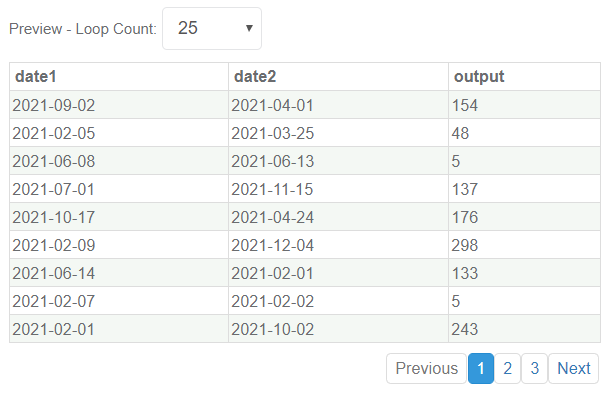# Description

The DateDeltaGen Generator computes the delta between two dates. The delta may be either days, months, years, or hours.

## Parameters

The following parameters may be configured for the DateDeltaGen Generator. Items with an asterisk* are required.

• conversion* - Defines how the result will be calculated. The options are:
• days
• months
• years
• hours

• formatOne* - Defines the format of the first date value being referenced.

• formatTwo* - Defines the format of the second date value being referenced.

• dateRefOne* - References an Attribute containing the value for the first date.

• dateRefTwo* - References an Attribute containing the value for the second date.## Example 1: Calculate the Delta (in days) Between 2 Randomly Generated Dates

For this example, two FlexibleDateRangeGen Generators have been linked and referenced by the DateDeltaGen Generator to generate 2 separate dates, which will be used to calculate the delta between them.## Sample Output

Conversion is days in the example below: